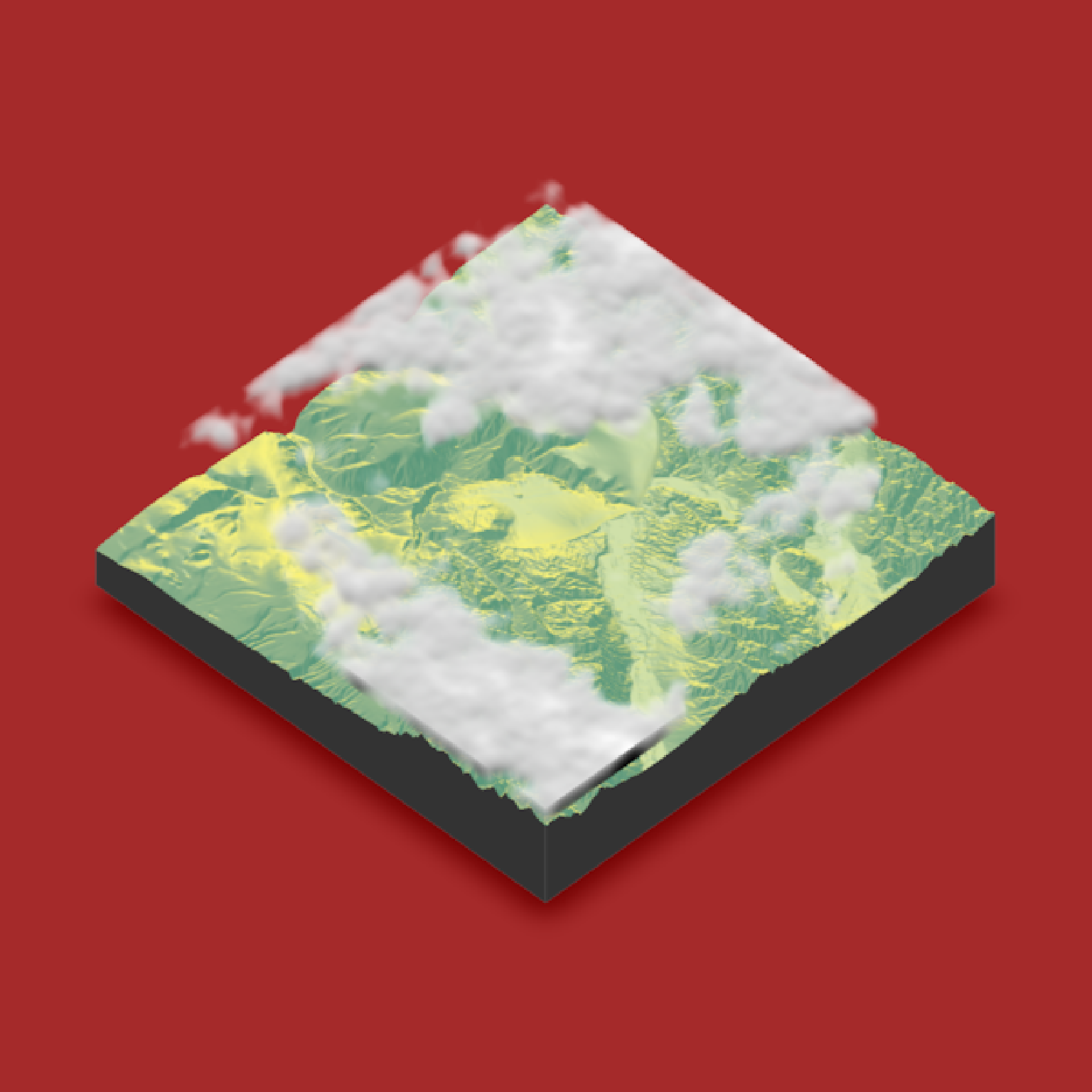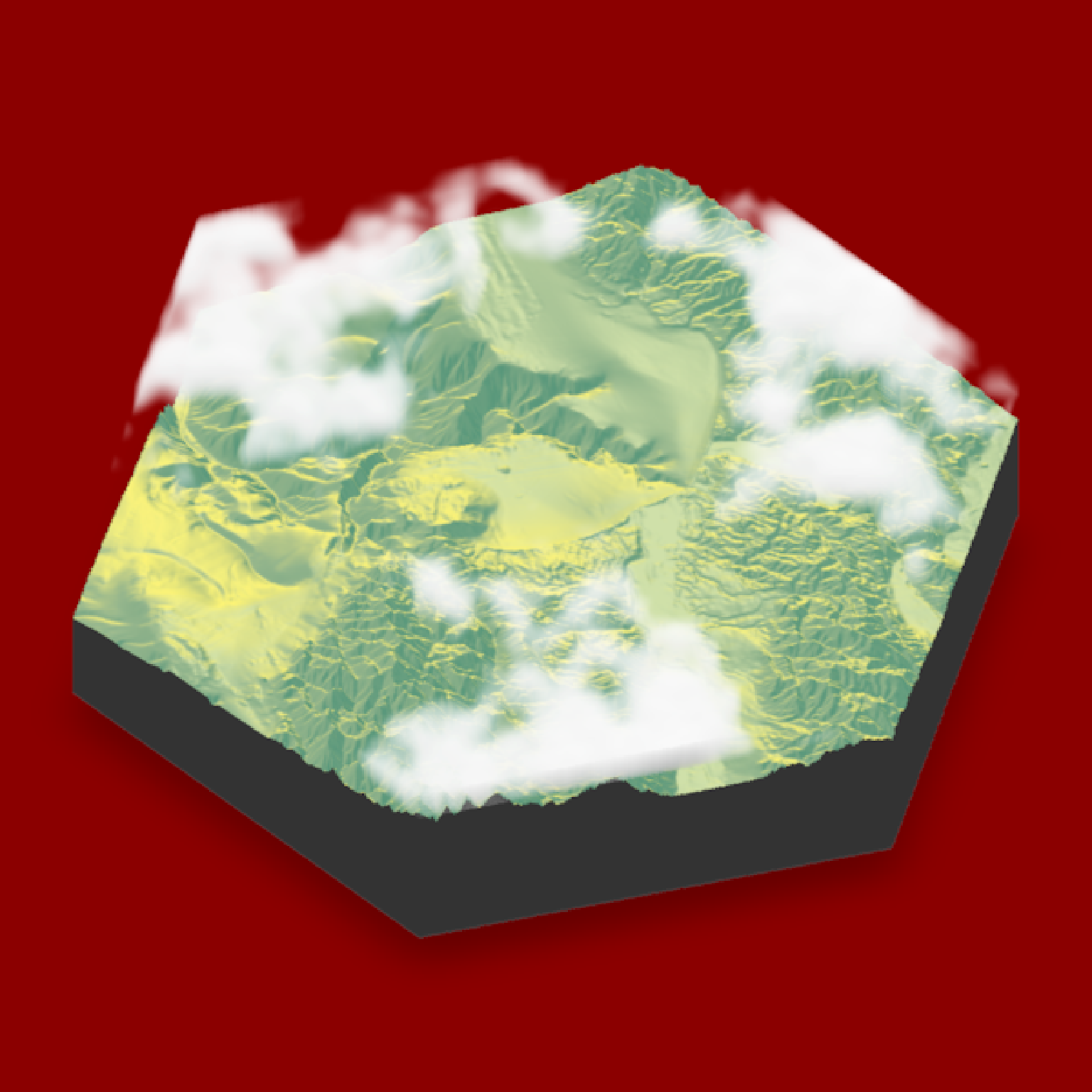Render a 3D floating cloud layer of the map.

Note: Underlying layers with transparency can cause rendering issues in rgl.

render_clouds(
heightmap,
start_altitude = 1000,
end_altitude = 2000,
sun_altitude = 10,
sun_angle = 315,
time = 0,
cloud_cover = 0.5,
layers = 10,
offset_x = 0,
offset_y = 0,
scale_x = 1,
scale_y = 1,
scale_z = 1,
frequency = 0.005,
fractal_levels = 16,
attenuation_coef = 1,
seed = 1,
zscale = 1,
baseshape = "rectangle",
clear_clouds = FALSE
)

## Arguments

heightmap

A two-dimensional matrix, where each entry in the matrix is the elevation at that point. This is used by render_clouds() to calculate the regions the clouds should be rendered in.

start_altitude

Default 1000. The bottom of the cloud layer.

end_altitude

Default 2000. The top of the cloud layer.

sun_altitude

Default 90. The angle, in degrees (as measured from the horizon) from which the light originates.

sun_angle

Default 315 (NW). The angle, in degrees, around the matrix from which the light originates. Zero degrees is North, increasing clockwise

time

Default 0. Advance this to make the clouds evolve and change in shape.

cloud_cover

Default 0.5. The percentage of cloud cover.

layers

Default 10. The number of layers to render the cloud layer. The default is layers/(start_altitude - end_altitude).

offset_x

Default 0. Change this to move the cloud layer sideways.

offset_y

Default 0. Change this to move the cloud layer backwards and forwards.

scale_x

Default 1. Scale the fractal pattern in the x direction.

scale_y

Default 1. Scale the fractal pattern in the y direction.

scale_z

Default 1. Scale the fractal pattern in the z (vertical) direction. (automatically calculated). Scale the fractal pattern in the z (vertical) direction.

frequency

Default 0.005. The base frequency of the noise used to calculate the fractal cloud structure.

fractal_levels

Default 16. The fractal dimension used to calculate the noise. Higher values give more fine structure, but take longer to calculate.

attenuation_coef

Default 1. Amount of attenuation in the cloud (higher numbers give darker shadows). This value is automatically scaled to account for increasing the number of layers.

seed

Default 1. Random seed used to generate clouds.

zscale

Default 1. The ratio between the x and y spacing (which are assumed to be equal) and the z axis. For example, if the elevation levels are in units of 1 meter and the grid values are separated by 10 meters, zscale would be 10.

baseshape

Default rectangle. Shape of the base. Options are c("rectangle","circle","hex").

clear_clouds

Default FALSE. Clears all existing floating layers on the visualization.

## Value

Adds a 3D floating cloud layer to the map. No return value.

## Examples

if(rayshader:::run_documentation()) {
#Render a cloud layer over Monterey Bay
montereybay  %>%
plot_3d(montereybay,background="brown",zscale=50)

#Render some clouds
render_clouds(montereybay, zscale=50)
render_snapshot()
}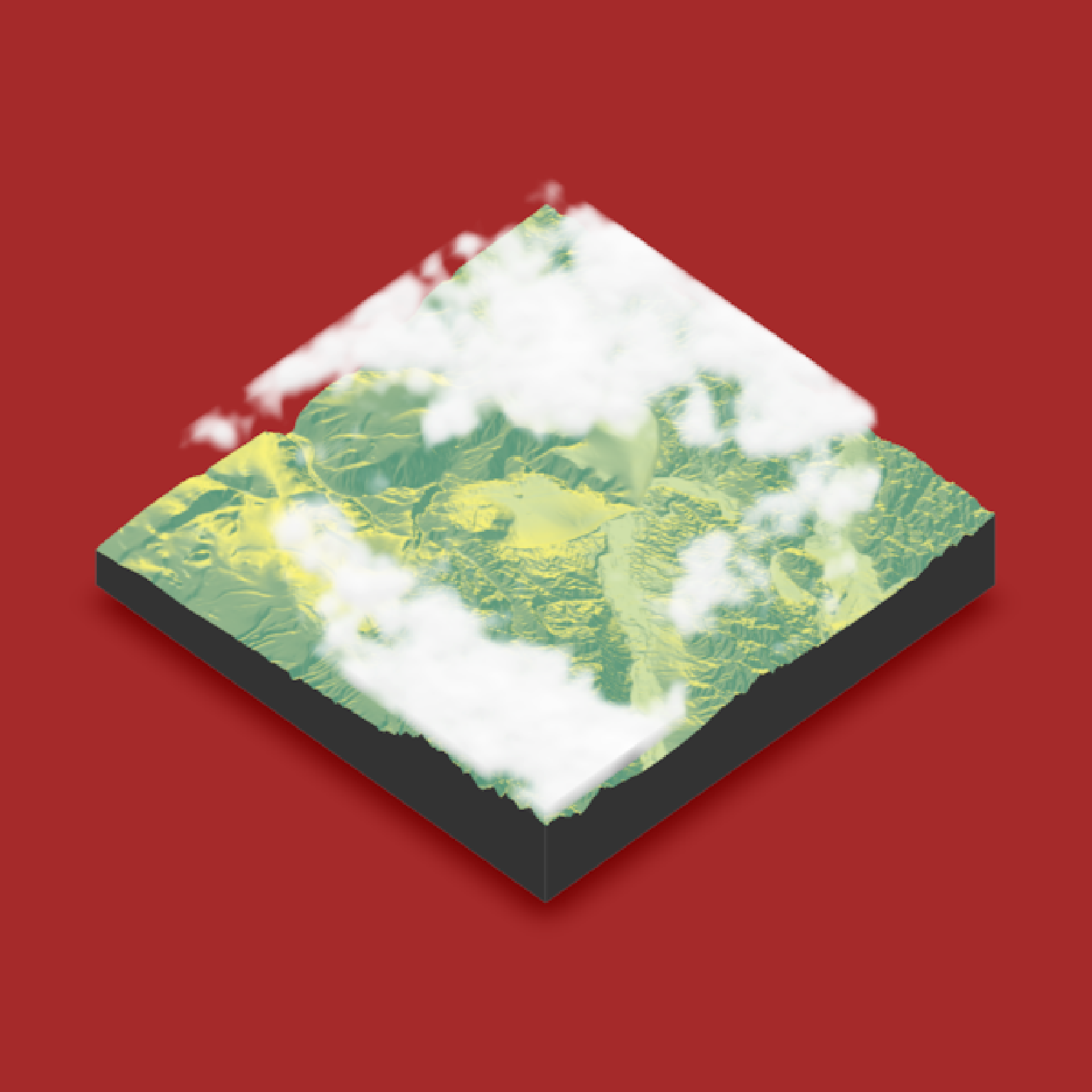#Change the seed for a different set of clouds and add cloud shadows on the ground
montereybay  %>%
plot_3d(montereybay,background="brown",zscale=50)
render_camera(theta=-65, phi = 25, zoom = 0.45, fov = 80)
render_clouds(montereybay, zscale=50, seed=2, clear_clouds = T)
render_snapshot()
}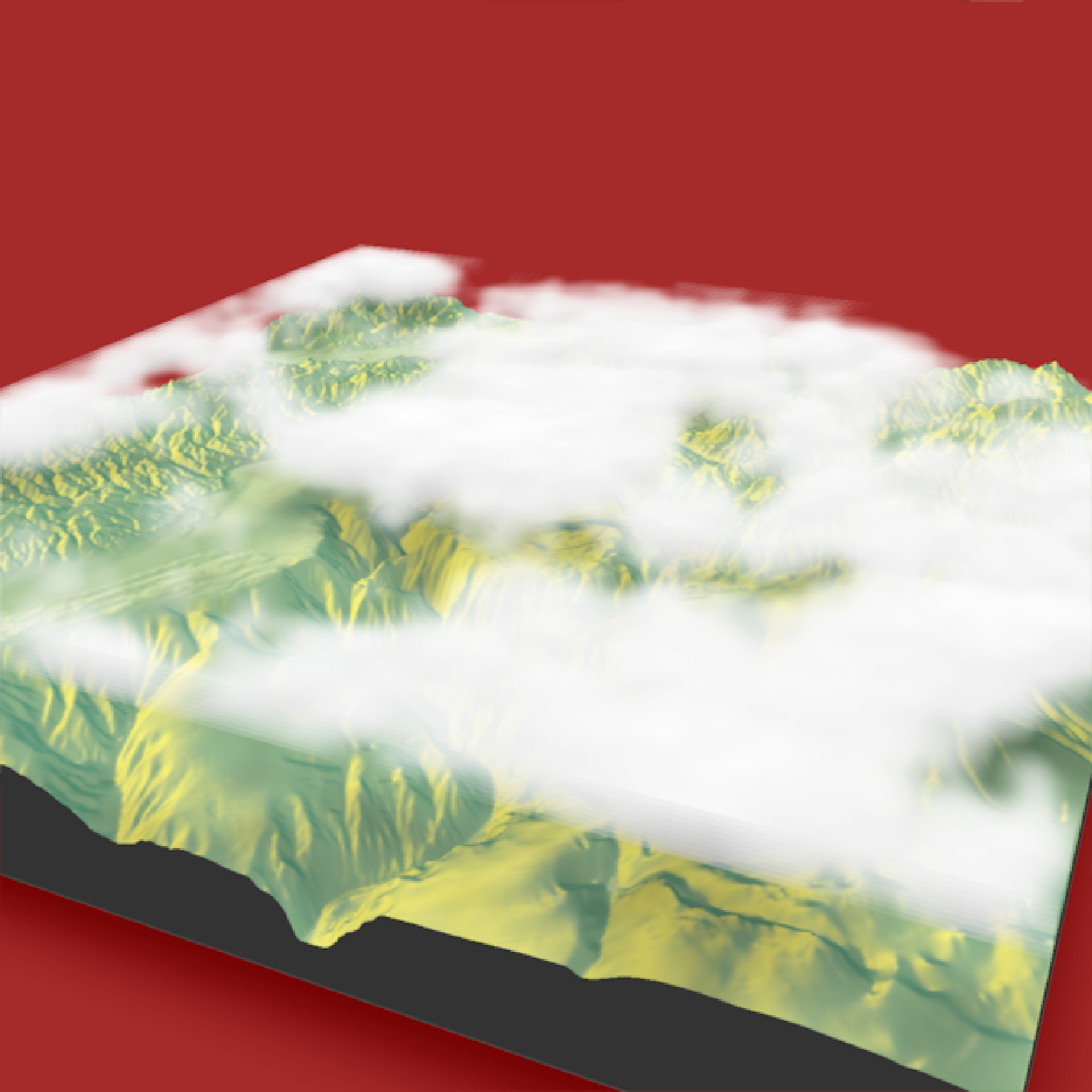montereybay  %>%
plot_3d(montereybay,background="brown",zscale=50)

#Lower the frequency for larger, smoother clouds
render_clouds(montereybay, zscale=50, frequency = 0.001, clear_clouds = T)
render_snapshot()
}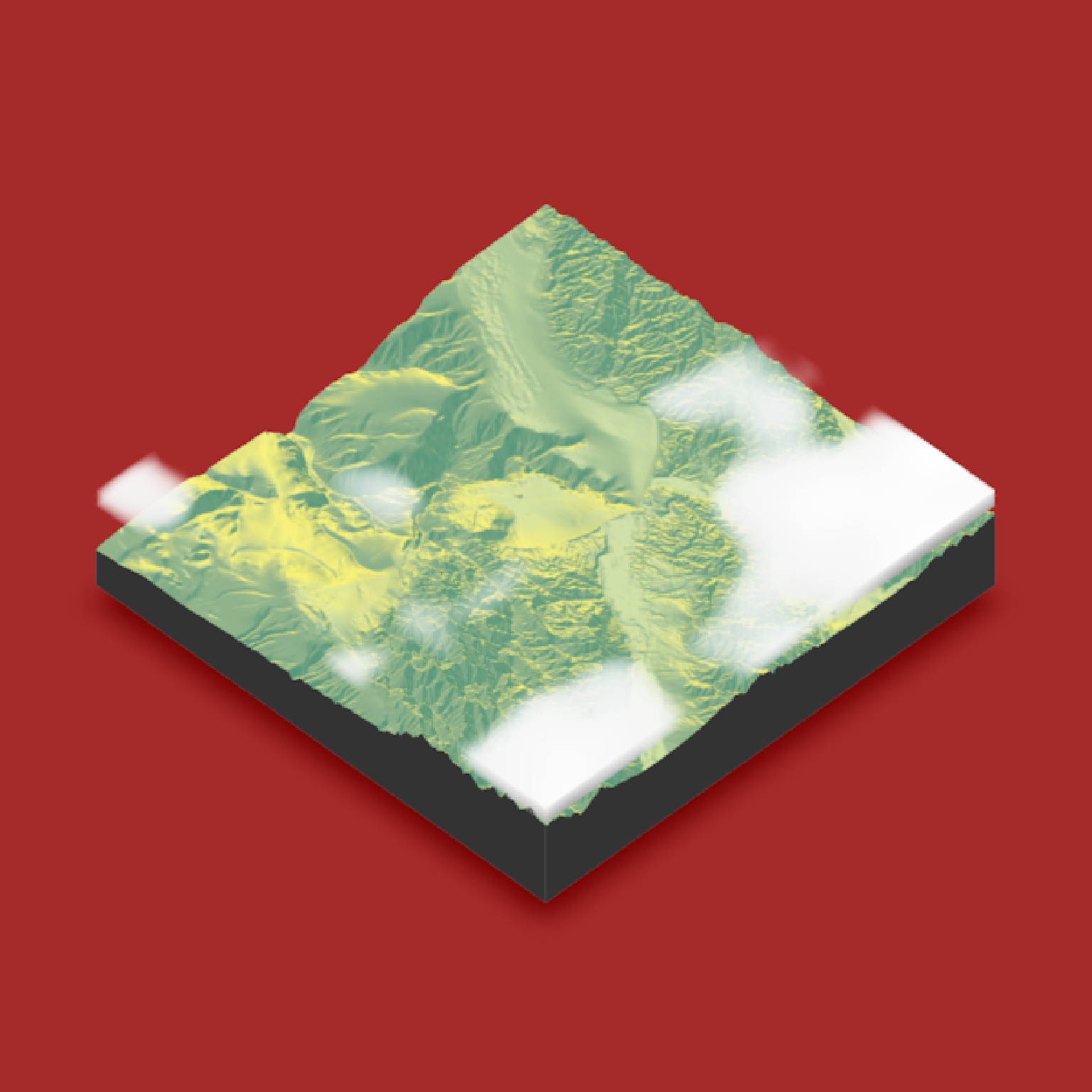#Increase the frequency for more broken clouds
render_clouds(montereybay, zscale=50, frequency = 0.05, clear_clouds = T)
render_snapshot()
}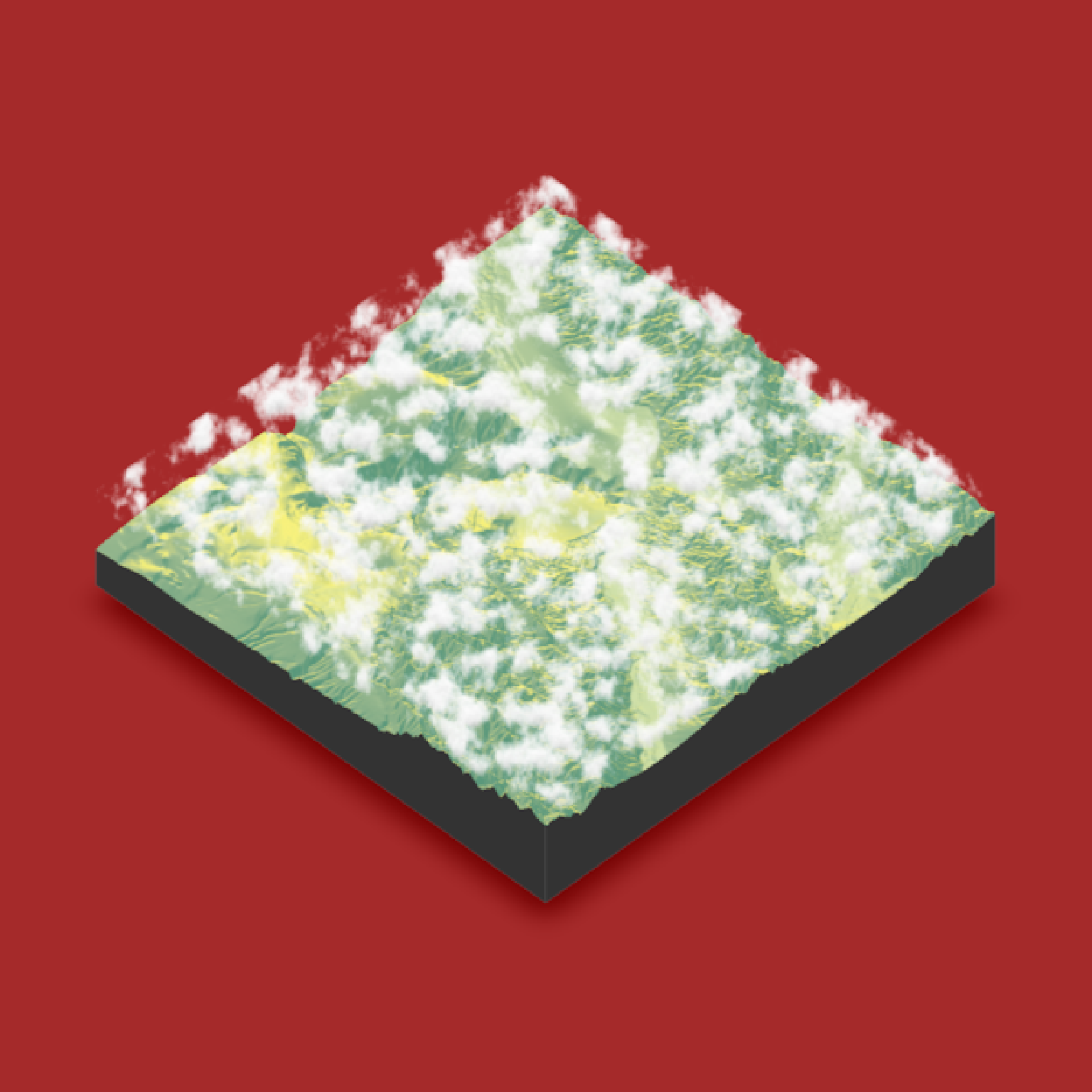#Increase the fractal level for fluffier, bumpier clouds
render_clouds(montereybay, zscale=50, fractal_levels = 32, clear_clouds = T)
render_snapshot()
}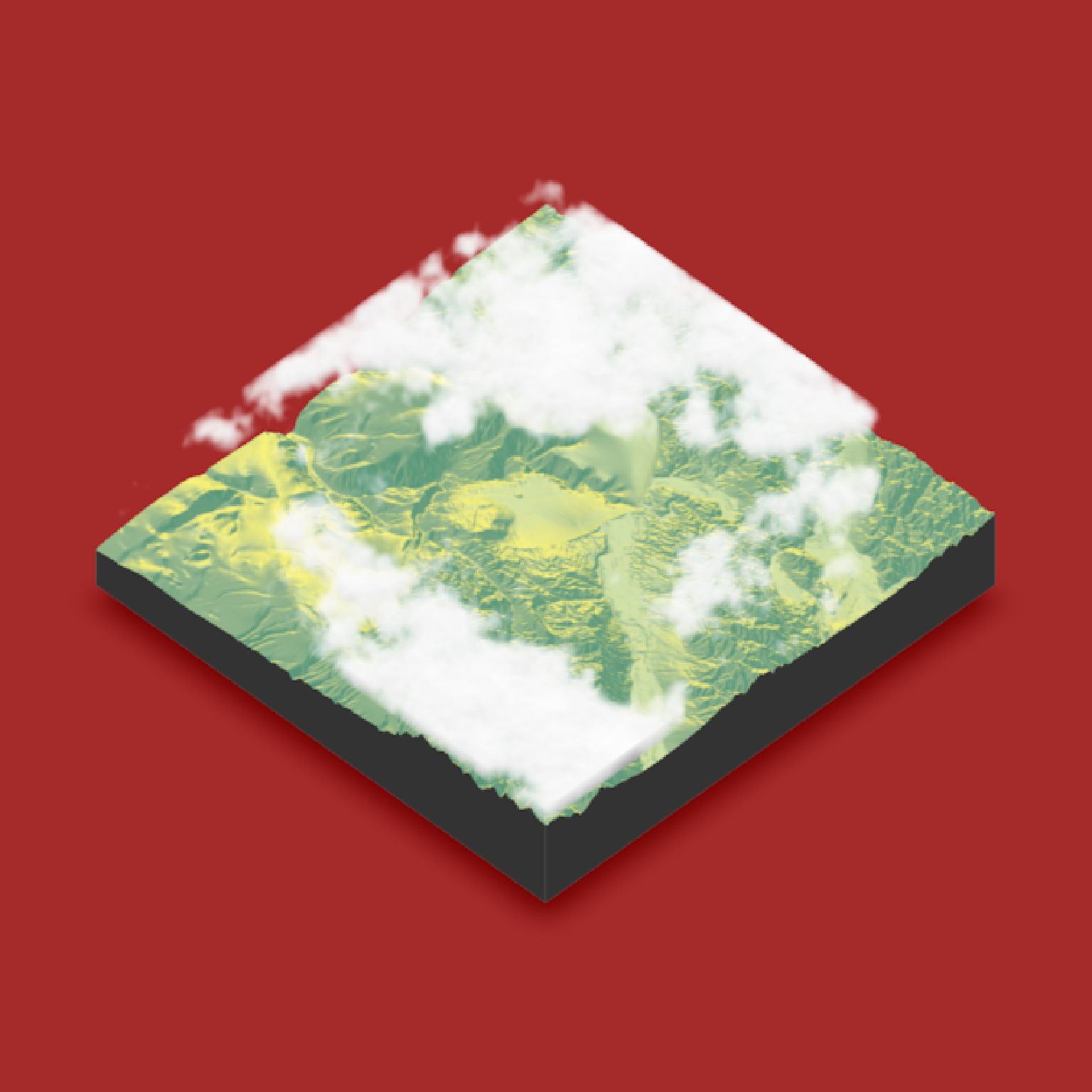#Decrease the fractal level for more smoother, continuous clouds
render_clouds(montereybay, zscale=50, fractal_levels = 4, clear_clouds = T)
render_snapshot()
}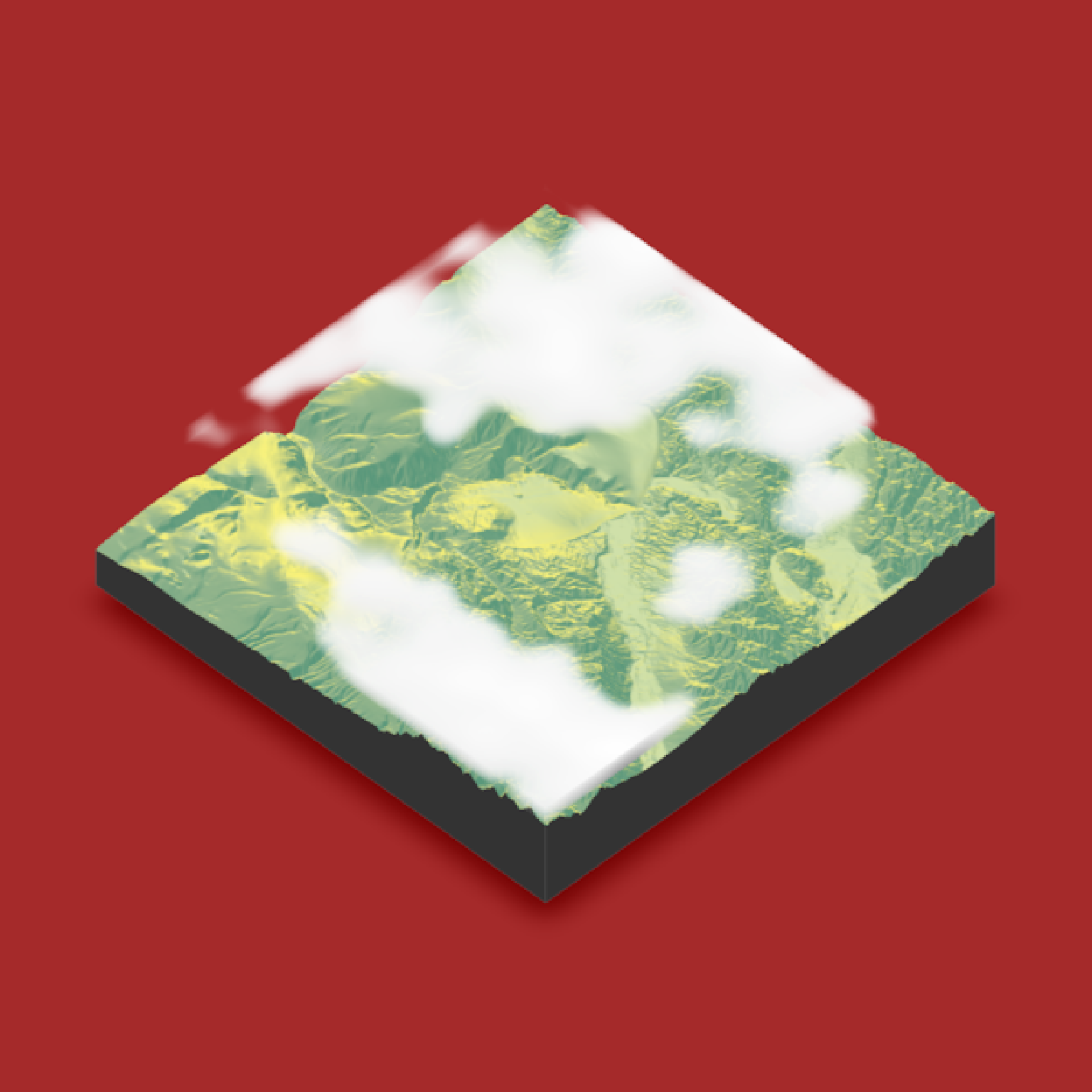#Increase the cloud cover
render_clouds(montereybay, zscale=50, cloud_cover=0.8, clear_clouds = T)
render_snapshot()
}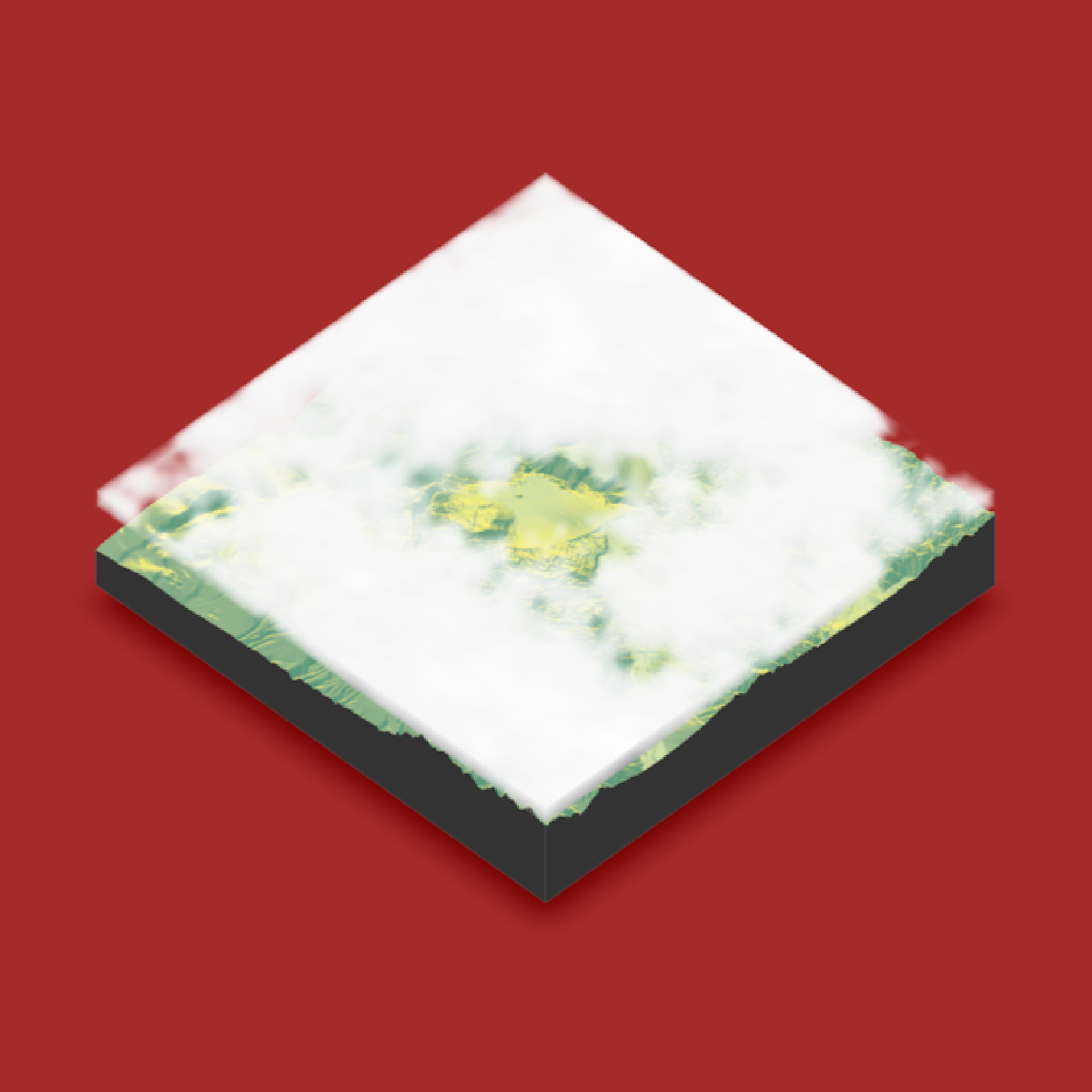#Decrease the cloud cover
render_clouds(montereybay, zscale=50, cloud_cover=0.2, clear_clouds = T)
render_snapshot()
}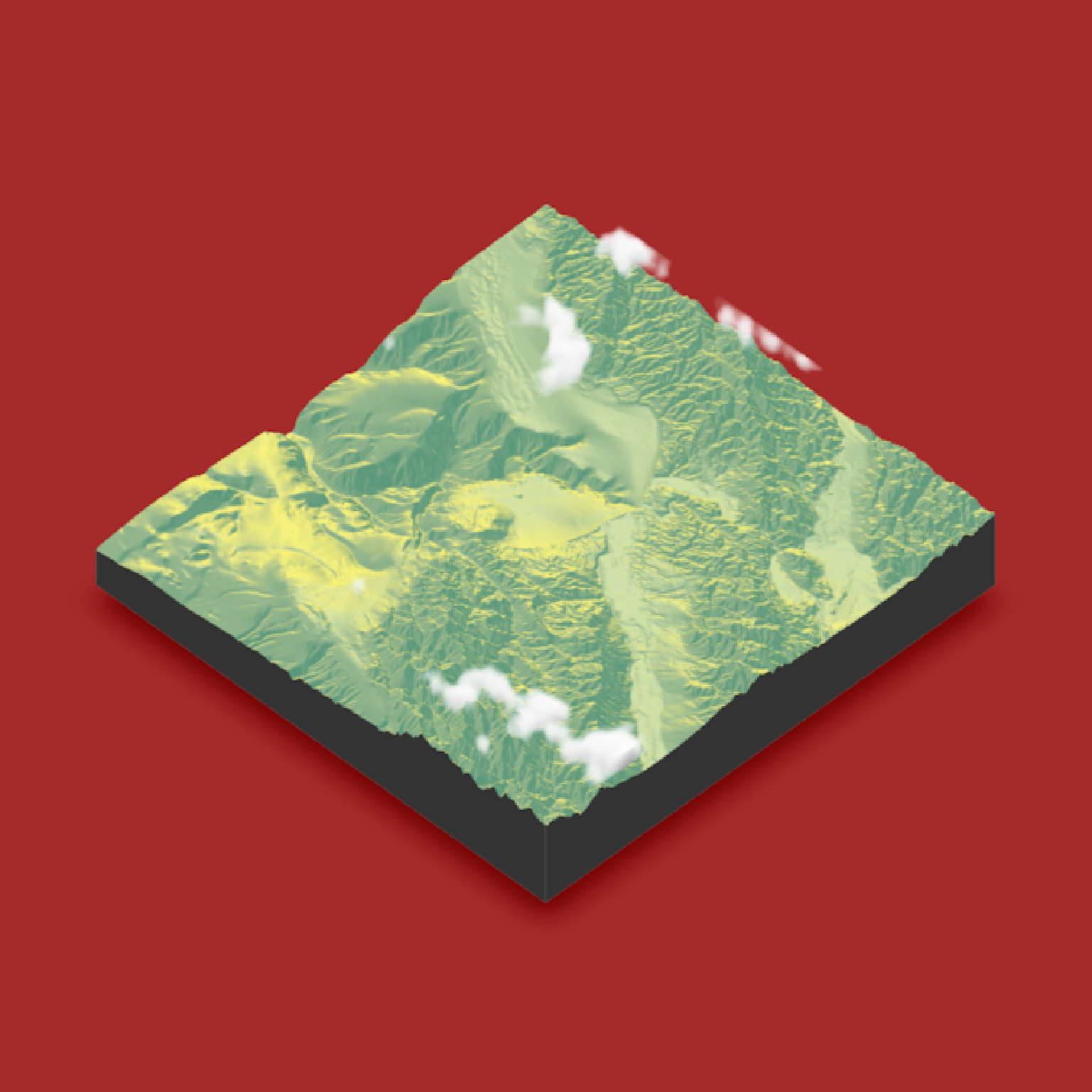#Change the altitude range of the clouds
render_clouds(montereybay,zscale=50,start_altitude=2000,end_altitude = 4000, clear_clouds = T)
render_snapshot()
}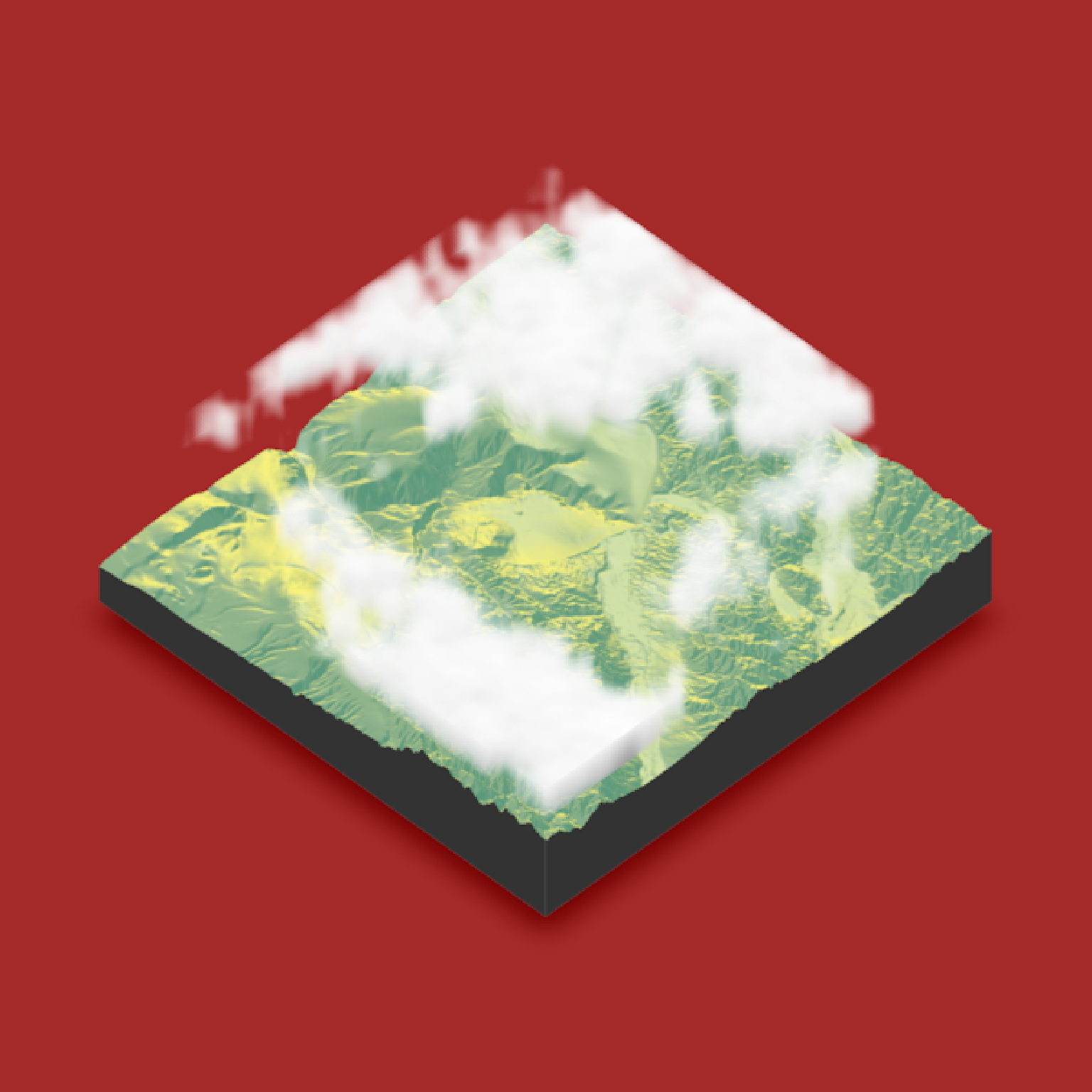#Increase the number of layers
render_clouds(montereybay, zscale=50,start_altitude=2000,end_altitude = 4000, layers = 20,
clear_clouds = T)
render_snapshot()
}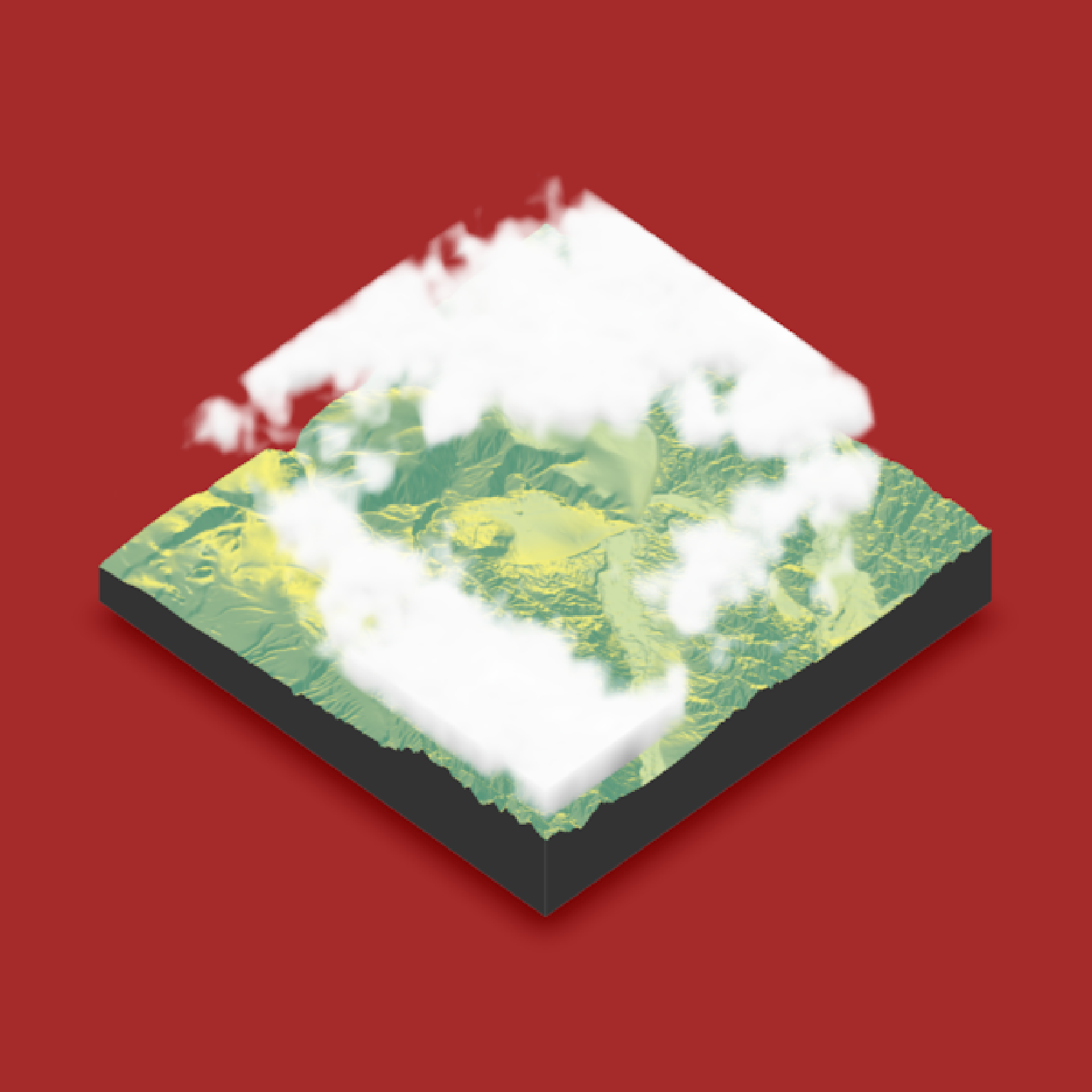#Change the sun angle and altitude, and increase the attenuation for darker clouds
render_clouds(montereybay,zscale=50,sun_angle=45, sun_altitude= 5, attenuation_coef = 5,
clear_clouds = T)
render_snapshot()
}